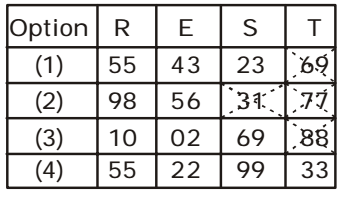## Grid and Matrices

#### Grid and Matrices

1. A word is represented by only one set of numbers as given in any one of the alternatives. The sets of numbers given in the alternatives are represented by two classes of alphabets as in two matrices given below. The columns and rows of Matrix I are numbered from 0 to 4 and that of Matrix II are numbered from 5 to 9. A letter from these matrices can be represented first by its row and next by its column, e.g., ‘A’ can be represented by 55, 67, 86, etc and ‘R’ can be represented by 04, 23, 30, etc. Identify the set for the word DOOR.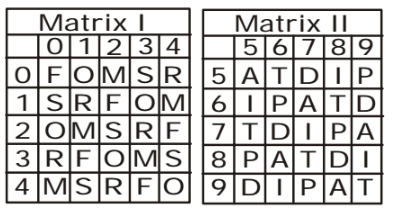1. 69, 44, 20, 43
2. 76, 01, 44, 24
3. 95, 20, 44, 12
4. 57, 13, 32, 23

1. As per given above matrix I and II , we can see that
D ⇒ 57, 69, 76, 88, 95
O ⇒ 01, 13, 20, 32, 44
R ⇒ 04, 11, 23, 30, 42

##### Correct Option: D

As per given above matrix I and II , we can see that
D ⇒ 57, 69, 76, 88, 95
O ⇒ 01, 13, 20, 32, 44
R ⇒ 04, 11, 23, 30, 42
As shown in given figure , the set for the word DOOR will be 57, 13, 32, 23 .From matrix , option ( 4 ) is correct .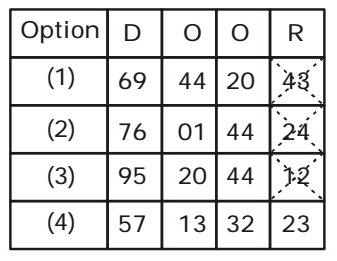1. A word is represented by only one set of numbers as given in any one of the alternatives. The sets of numbers given in the alternatives are represented by two classes of alphabets as in two matrices given below. The columns and rows of Matrix I are numbered-from 0 to 4 and that of Matrix II are numbered from 5 to 9. A letter from these matrices can be represented first by its row and next by its column, e.g., ‘B’ can be represented by 01, 31 etc. and ‘P’ can be represented by 67, 75 etc. Similarly, you have to identify the set for the word CARD.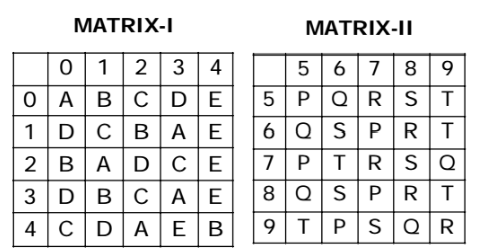1. 32, 00, 56, 10
2. 40, 21, 68, 44
3. 11, 33, 57, 22
4. 02, 42, 77, 20

1. From above given matrices I and II , we get different values for word CARD -
C ⇒ 02, 11, 23, 32, 40
A ⇒ 00, 13, 21, 33, 42
R ⇒ 57, 68, 77, 88, 99
D ⇒ 03, 10, 22, 30, 41

##### Correct Option: C

From above given matrices I and II , we get different values for word CARD -
C ⇒ 02, 11, 23, 32, 40
A ⇒ 00, 13, 21, 33, 42
R ⇒ 57, 68, 77, 88, 99
D ⇒ 03, 10, 22, 30, 41
As shown in given figure , all the values for word CARD are matched in only option ( 3 ) for every letter . So , the set for the word CARD will be 11, 33, 57, 22 .From matrix , option ( 3 ) is correct .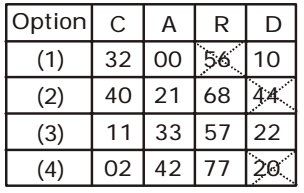1. A word is represented by only one set of numbers as given in any one of the alternatives. The sets of numbers given in the alternatives are represented by two classes of alphabets as in two matrices given below. The columns and rows of Matrix I are numbered from 0 to 4 and that of Matix II are numbered from 5 to 9. A letter from these matrices can be represented first by its row and next by its column, eg, ‘A’ can be represented by 40, 01, 13, 32 ‘etc., and ‘N’ can be represented by 56, 68, 89 etc. Similarly, you have to identify the set for the word given below:
SIX-KIDS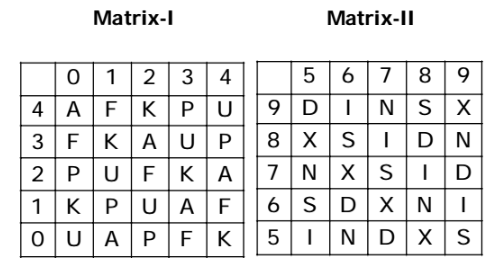1. 86, 87, 99 – 40, 41, 86, 64
2. 98, 96, 85 – 42, 78, 88, 77
3. 77, 69, 76 – 22, 95, 28, 31
4. 65, 55, 67 – 05, 25, 91, 40

1. As per above given matrices I and II , we can see that
S ⇒ 98, 86, 77, 65, 59
I ⇒ 96, 87, 78, 69, 55
X ⇒ 99, 85, 76, 67, 58
K ⇒ 42, 31, 23, 10, 04
D ⇒ 95, 88, 79, 66, 57

##### Correct Option: B

As per above given matrices I and II , we can see that
S ⇒ 98, 86, 77, 65, 59
I ⇒ 96, 87, 78, 69, 55
X ⇒ 99, 85, 76, 67, 58
K ⇒ 42, 31, 23, 10, 04
D ⇒ 95, 88, 79, 66, 57
As shown in given figure , all the values for word SIX-KIDS are matched in only option ( 2 ) for every letter . So , the set for the word SIX-KIDS will be 98, 96, 85 – 42, 78, 88, 77 .From matrix , option ( 2 ) is correct .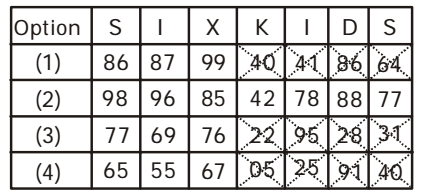1. A word is represented by only one set of numbers as given in any one of the alternatives. The sets of numbers given in the alternatives are represented by two classes of alphabets as in two matrices given below. The columns and rows of Matrix Iare numbered from 0 to 4 and 2 to 6 respectively and that of Matrix II are numbered from 2 to 6 and 7 to 0 respectively. A letter from these matrices can be represented first by its row and next by its column, e.g., ‘H’ can be represented by 04, 25, 32, etc., and ‘N’ can be represented by 21, 40, 59, etc. Similarly, you have to identify the set for the word given below:
YEAR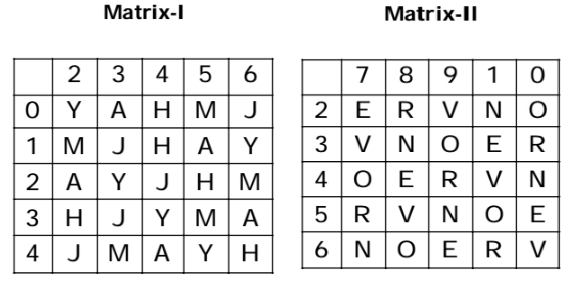1. 23, 27, 15, 61
2. 16, 38, 15, 30
3. 34, 31, 32, 28
4. 45, 50, 36, 29

1. As per above given matrices I and II , we can see that
Y ⇒ 02, 16, 23, 34, 45
E ⇒ 27, 31, 48, 50, 69
A ⇒ 03, 15, 22, 36, 44
R ⇒ 28, 30, 49, 57, 61

##### Correct Option: A

As per above given matrices I and II , we can see that
Y ⇒ 02, 16, 23, 34, 45
E ⇒ 27, 31, 48, 50, 69
A ⇒ 03, 15, 22, 36, 44
R ⇒ 28, 30, 49, 57, 61
From given matrix it is clear that all the values for word YEAR are matched in only option ( 1 ) for every letter . So , the set for the word YEAR will be 23, 27, 15, 61 .From matrix , option ( 1 ) is correct .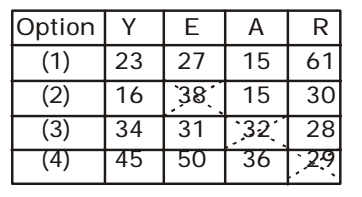1. A word is represented by only one set of numbers as given in any one of the alternatives. The sets of numbers given in the alternatives are represented by two classes of alphabets as in two matrices given below. The columns and rows of Matrix I are numbered from 0 to 4 and that of Matrix II are numbered from 5 to 9. A letter from these matrices can be represented first by its row and next by its column, e.g. ‘A’ can be represented by 03, 34, 86, etc. and ‘N’ can be represented by 12, 65, 79, etc. Similarly, you have to identify the set for the given word.
REST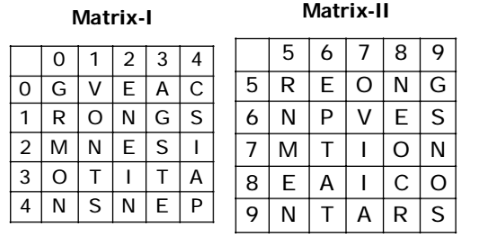1. 55, 43, 23, 69
2. 98, 56, 31, 77
3. 10, 02, 69, 88
4. 55, 22, 99, 33

1. As per above given matrices I and II , we can see that
R ⇒ 10, 55, 98
E ⇒ 02, 22, 43, 56, 68, 85
S ⇒ 14, 23, 41, 69, 99
T ⇒ 31, 33, 76, 96

##### Correct Option: D

As per above given matrices I and II , we can see that
R ⇒ 10, 55, 98
E ⇒ 02, 22, 43, 56, 68, 85
S ⇒ 14, 23, 41, 69, 99
T ⇒ 31, 33, 76, 96
As shown in given figure , all the values for word REST are matched in only option ( 4 ) for every letter . So , the set for the word REST will be 55, 22, 99, 33 .From matrix , option ( 4 ) is correct .